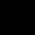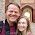## Thursday, December 5, 2013

### Incidental Parameters Problem with Binary Response Data and Unobserved Individual Effects

It is a well known problem that in some models as the number of observations becomes large, econometric estimators fail to converge on consistent estimators.  The leading case of this is when estimating a binary response model with panel data with potential "fixed effects" correlated with the explanatory variables appearing in the population.

One method that is typically implemented by researchers is to observe inviduals or organizations over multiple periods of time.  This is called panel data.  Within the context of panel data it is often assumed unobserved effects: genetics, motivation, business structure, and whatever other unobservables that might be correlated with the explanatory variables are unchanging over time.

If we do then it is relatively easy to remove the effect of unobservables from our analysis.  In my previous post I demonstrate 3 distinct but equivalent methods for accomplishing this task when our structural model is linear.

However, when our model is a binary response variables (graduate from college or not, get married or not, take the job or not, ect.) it is usually no longer logically consistent to stick with a linear model.

In addition, not all of our remidies which worked for the linear model provide consistent estimators for non-linear models.  Let us see this in action.

First we will start with generating the data as we did in the December 4th post.

```# Let's say: x = x.base + fe

set.seed(2)

nperson <- 500 # Number of persons
nobs <- 5      # Number of observations per person

fe.sd <- 1 # Spefify the standard deviation of the fixed effed
x.sd  <- 1 # Specify the base standard deviation of x

# First generate our data using the time constant effects
constantdata <- data.frame(id=1:nperson, fe=rnorm(nperson))

# We expand our data by nobs
fulldata <- constantdata[rep(1:nperson, each=nobs),]

# Add a time index, first define a group apply function
# that applies by group index.
gapply <- function(x, group, fun) {
returner <- numeric(length(group))
for (i in unique(group))
returner[i==group] <- get("fun")(x[i==group])
returner
}

# Using the generalized apply function coded above
fulldata\$t <- gapply(rep(1,length(fulldata\$id)),
group=fulldata\$id,
fun=cumsum)

# Now we are ready to caculate the time variant xs
fulldata\$x <- fulldata\$fe + rnorm(nobs*nperson)

# This is were our simulation diverges from a linear model.

# Let us define the linear component as:
lc <- .5*fulldata\$x + .5*fulldata\$fe

# Now we will define the logit probability as
fulldata\$pl <- exp(lc)/(1+exp(lc))

# Now we define the probit probability as
fulldata\$pp <- pnorm(lc)

cor(fulldata\$pl,fulldata\$pp)

plot(fulldata\$pl,fulldata\$pp, main="Probit and Logit Probabilities",
xlab="Logit", ylab="Probit")

plot(sort(lc), sort(fulldata\$pl), main="Probit and Logit Probabilities",
ylab="P(y=1|x,a)", xlab="xb+a",
type="l", lwd=2, lty=3)
lines(sort(lc), sort(fulldata\$pp), lwd=2, lty=2)
legend(-3.3,.8,c("logit","probit"), lty=c(3,2), lwd=2) ```

`# We can see the probit tends to have a shorter range in which the `
`# action is happening. `
```
# We should really think of the probit and the logit as now
# two different sets of data.  Now let's generate out outcomes.
fulldata\$yl <- fulldata\$pl>runif(nobs*nperson)
fulldata\$yp <- fulldata\$pp>runif(nobs*nperson)

# Let's try to estimate our parameters with the logit.
glm(yl ~ x, data = fulldata, family = "binomial")

# We can see our estimator is upwards biased as we expect.

# Now we will try to inlcude fixed effects as if we were not
# aware of the incidental parameters problem.
glm(yl ~ x+factor(id), data = fulldata, family = "binomial")

# We can see, that including a matrix of dummy variables
# seems to actually make our estimator worse.

# Instead let's try the remaining fix that is available to us
# from the previous post listing 3 fixes.

# We will include an average level of the explanatory variable
# for each individual.  This is referred to as the
# Chamberlain-Munlak device.
fulldata\$xmean <- ave(fulldata\$x, group=fulldata\$id)

glm(yl ~ x+xmean, data = fulldata, family = "binomial")
# We can see that including an average effect significantly
# reduces the inconsistency in the estimator.

# Now, let's see what happens if we do the same things in the
# probit model.
glm(yp ~ x, data = fulldata, family = binomial(link = "probit"))
# Probit experiences similar upward bias to that of the logit.

glm(yp ~ x+factor(id), data = fulldata,

glm(yp ~ x+xmean, data = fulldata, family = binomial(link = "probit"))
# Interestingly, including the Chamberlain-Munlak device in the probit
# though theoretically inconsistent does seem produce estimates
# comparably good as including the device with the logit at least
# in the sample sizes simulated here.```
Created by Pretty R at inside-R.org

1.I modeled the logit data with clogit(yl ~ x+strata(id),fulldata,method="approximate") (i.e., conditional logit) from the survival package expecting to get the same results as your glm model with factor(id) included. However, the coefficient estimate for x was 0.22 (0.03).

1.Hmm, well. I don't have any answers for you but I will hit the books and see what pops out.

2.Also, consider by() and ave() in base R as alternatives to your gapply() function.

1.Thank you for the suggestion. I tried using ave but could not get it to work for me since I needed the function to return a vector for each group rather than a scalar. I did not try the by command.

3.I always thank you for your positing! Hong from South Korea.

4.